Square Roots 3
Set 3 (10 Questions)
Students use exponents, powers, and roots, and use exponents in working with fractions
From Mr. Anker Tests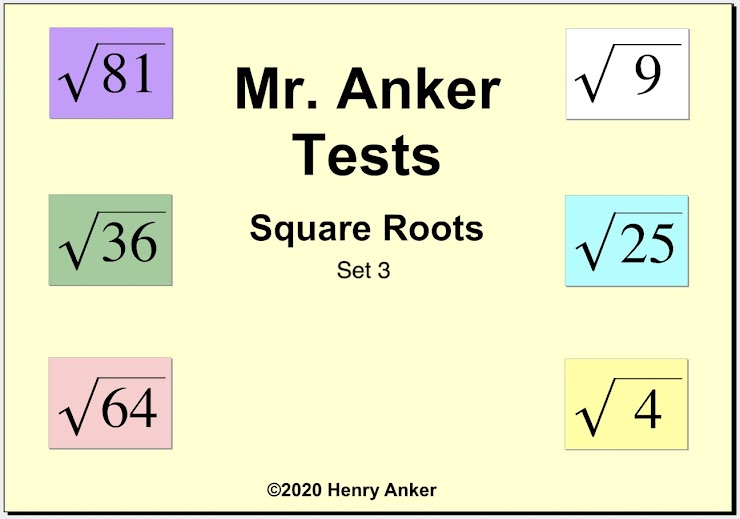Square Roots Help Slide 1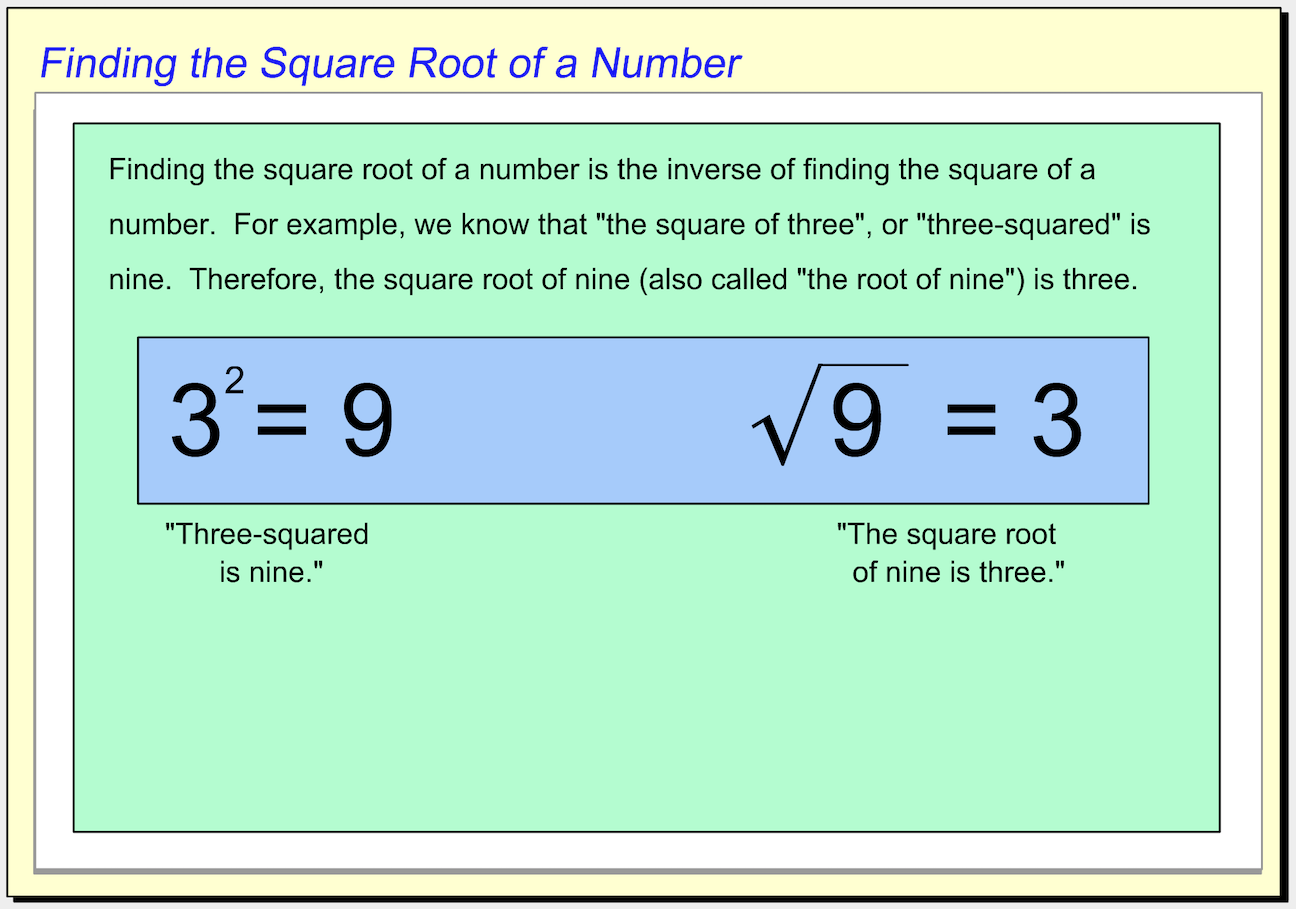Square Roots Help Slide 2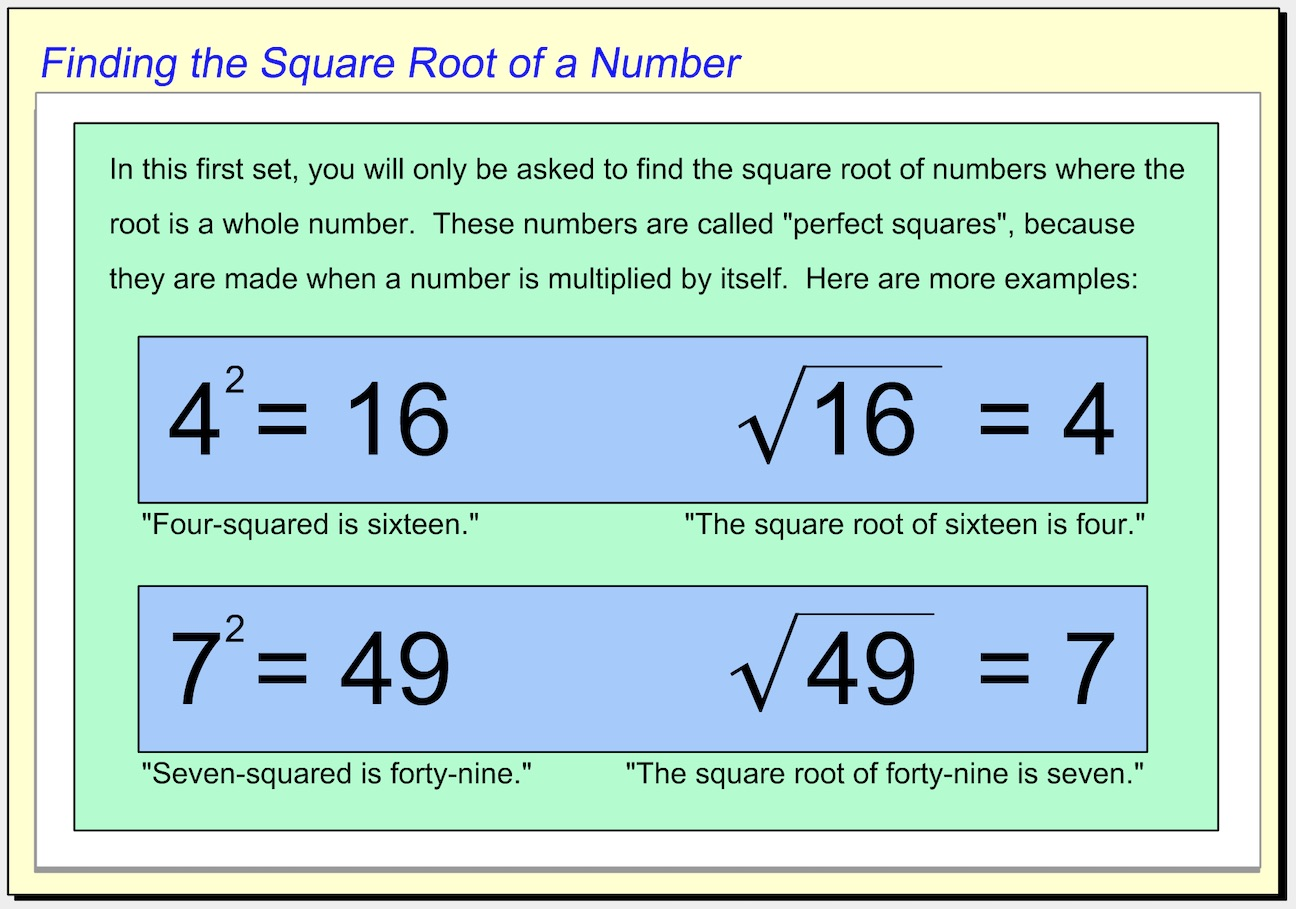Square Roots Help Slide 3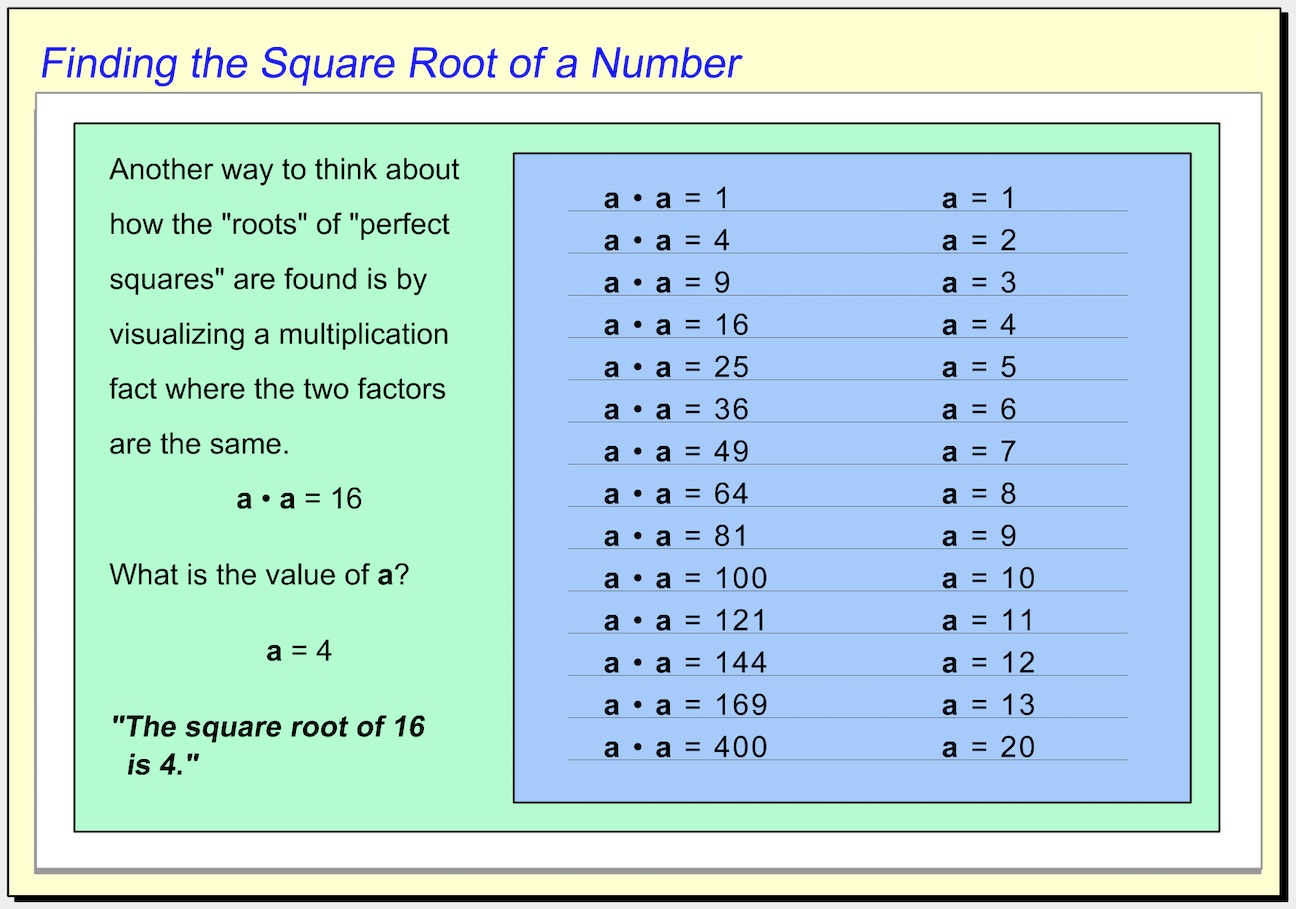Square Roots Help Slide 4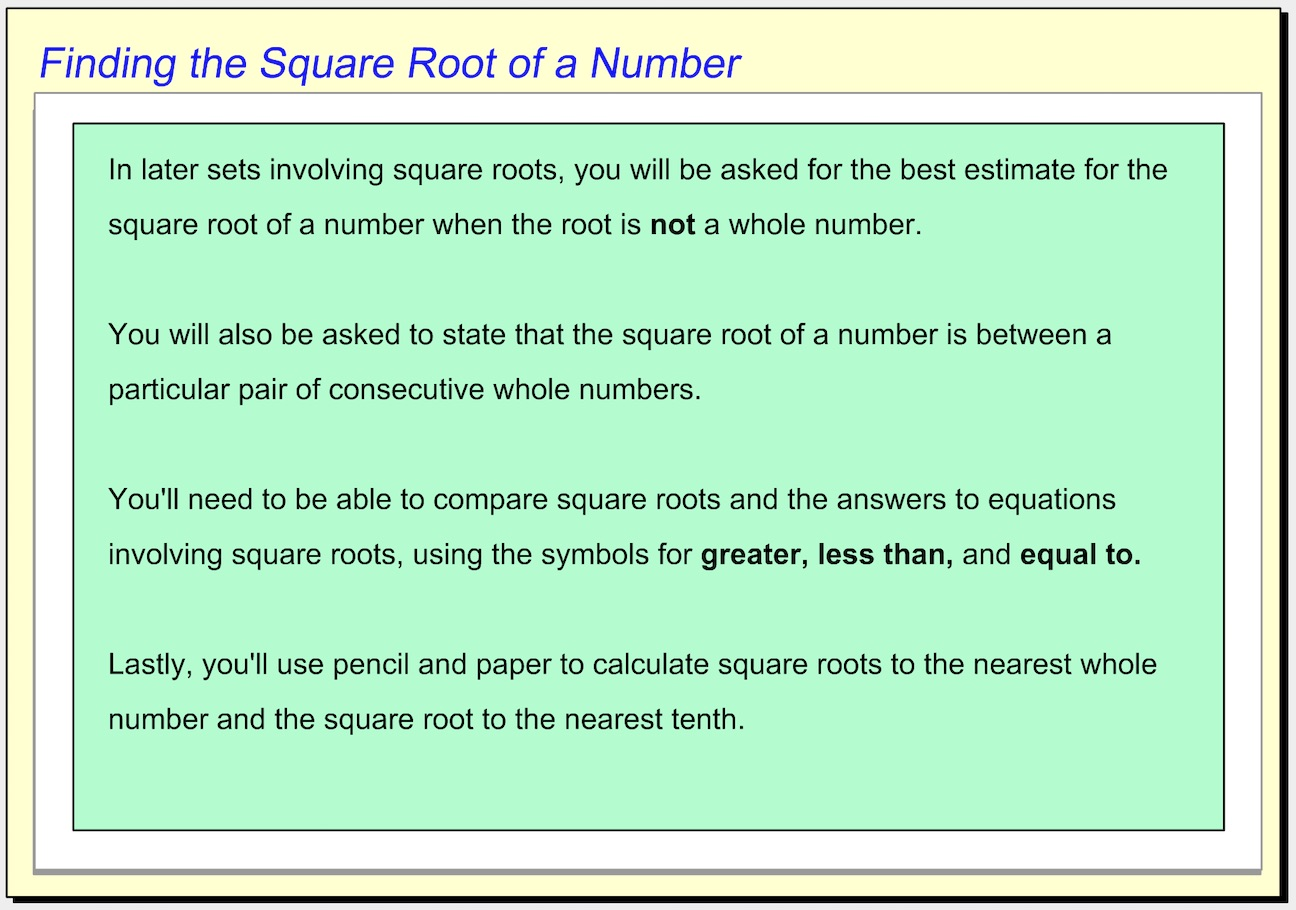1.  Which number goes in the box to make the equation true? *
1 point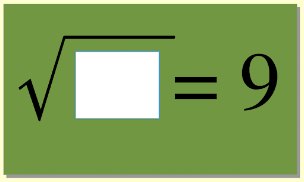2.  Which choice answers the question displayed? *
1 point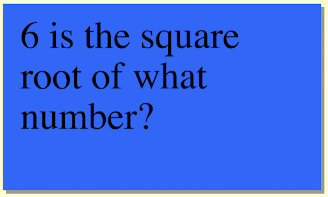3. Use the dropdown menu below to make this statement true: "Four squared minus seven is __________ the square root of 100." *
1 point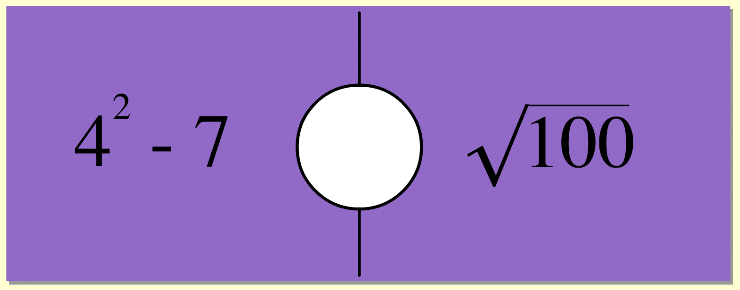4. On the line below, enter the equal value to this square root. *
1 point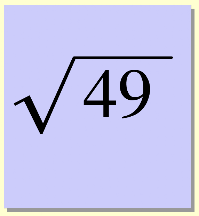5.  Which choice answers the question displayed? *
1 point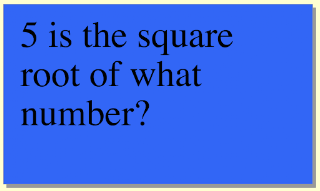6.  Use the dropdown menu below to make this statement true: "The square root of sixteen added to the square root of twenty-five is __________ the square root of eighty-one minus the square root of nine." *
1 point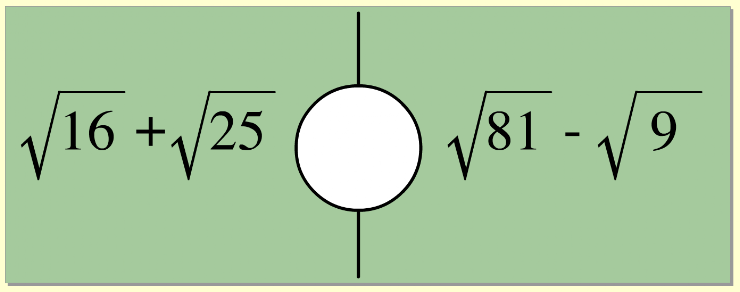7.  On the line below, enter the equal value to this square root. *
1 point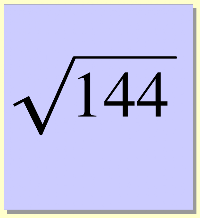8.  Use the dropdown menu below to make this statement true: "the square root of four times the square root of one hundred is __________ the square root of twenty-five times the square root of sixteen." *
1 point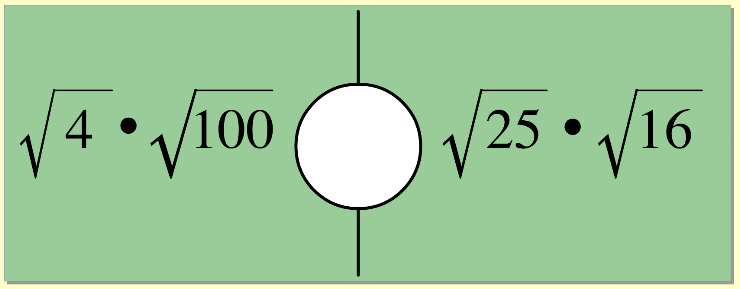9.  On the line below, enter the equal value as a whole number. *
1 point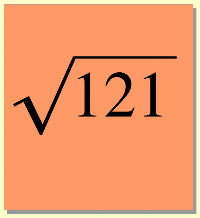10.  Which choice answers the question displayed? *
1 point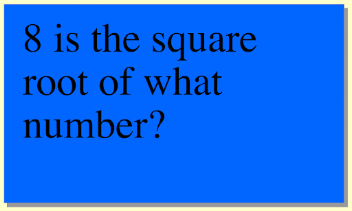Submit
Clear form